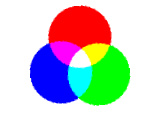# Log24

## Wednesday, December 4, 2002

### Wednesday December 4, 2002

Filed under: General,Geometry — m759 @ 11:22 PM

Symmetry and a Trinity

From a web page titled Spectra:

"What we learn from our whole discussion and what has indeed become a guiding principle in modern mathematics is this lesson:

Whenever you have to do with a structure-endowed entity  S try to determine its group of automorphisms, the group of those element-wise transformations which leave all structural relations undisturbed. You can expect to gain a deep insight into the constitution of S in this way. After that you may start to investigate symmetric configurations of elements, i.e., configurations which are invariant under a certain subgroup of the group of all automorphisms . . ."

— Hermann Weyl in Symmetry, Princeton University Press, 1952, page 144"… any color at all can be made from three different colors, in our case, red, green, and blue lights. By suitably mixing the three together we can make anything at all, as we demonstrated . . .

Further, these laws are very interesting mathematically. For those who are interested in the mathematics of the thing, it turns out as follows. Suppose that we take our three colors, which were red, green, and blue, but label them A, B, and C, and call them our primary colors. Then any color could be made by certain amounts of these three: say an amount a of color A, an amount b of color B, and an amount c of color C makes X:

X = aA + bB + cC.

Now suppose another color Y is made from the same three colors:

Y = a'A + b'B + c'C.

Then it turns out that the mixture of the two lights (it is one of the consequences of the laws that we have already mentioned) is obtained by taking the sum of the components of X and Y:

Z = X + Y = (a + a')A + (b + b')B + (c + c')C.

It is just like the mathematics of the addition of vectors, where (a, b, c ) are the components of one vector, and (a', b', c' ) are those of another vector, and the new light Z is then the "sum" of the vectors. This subject has always appealed to physicists and mathematicians."

— According to the author of the Spectra site, this is Richard Feynman in Elementary Particles and the Laws of Physics, The 1986 Dirac Memorial Lectures, by Feynman and Steven Weinberg, Cambridge University Press, 1989.

These two concepts — symmetry as invariance under a group of transformations, and complicated things as linear combinations (the technical name for Feynman's sums) of simpler things — underlie much of modern mathematics, both pure and applied.